# LOW POWER VLSI ARCHITECTURES FOR CRYPTOGRAPHIC ALGORITHMS

N/A
N/A
Protected

Share "LOW POWER VLSI ARCHITECTURES FOR CRYPTOGRAPHIC ALGORITHMS"

Copied!
134
0
0

Full text

This is to prove that the thesis titled "Low Power VLSI Architecture for Cryptographic Algorithms" submitted by BHOOPAL RAO GANGADARI, a Research Scholar in the Department of Electronics and Electrical Engineering, Indian Institute of Technology Guwahati, for the award of Ph.D. of Philosophy, is a record of original research work carried out by him under my supervision and direction. This is to certify that the thesis entitled "Low Power VLSI Architecture for Cryptographic Algorithms" submitted by BHOOPAL RAO GANGADARI submitted by me to the Indian Institute of Technology Guwahati for the award of the degree of Doctor of Philosophy is a work original research conducted. outside of me under the supervision of Prof. This thesis then characterizes a special class of Cellular Automata (CA) based architectures for the hardware implementation of the S-Box.

We have performed the security analysis of the proposed encryption algorithms using cryptographic properties such as non-linearity, strict avalanche criteria, correlation immunity bias and entropy. 29 3.4 Values ​​using cryptographic properties for different affine matrix of S-Box 29 3.5 FPGA Implementation of CFA for AES algorithm. 58 4.7 Hardware results of the proposed AES algorithm with RCA2-based S-Box 58 5.1 Values ​​obtained with cryptographic properties for ciphertext of HLCA.

## Introduction

The plain text is converted into cipher text using a secret key by a conventional encryption algorithm. In symmetric key ciphers, the secret key is shared between the intended sender and the recipient. Block encryption algorithms are widely used in the world of cryptography and work on a block of data for encryption and decryption.

The latest IEEE 802.15.6 standard for WBAN application has recommended a secret key size of 128 bits for the AES algorithm resulting in 10 rounds of transformation [3, 6]. The number of rounds of the feistel network is determined by the length of the secret key. The latest IEEE 802.15.6 standard for WBAN application has recommended a secret key size of 128 bits for the Camellia algorithm resulting in 18 rounds of the feistel network [3, 6].

## Motivation

In order to meet the requirements of the IEEE 802.15.6 WBAN standard, in this thesis we have exploited the concept of CA to obtain a low-energy architecture for the AES and Camellia algorithms. Since the implementation of the CA structure on hardware uses simple logic gates, the S-Box can be realized on hardware using CA to achieve low-power architectures suitable for WBAN applications.

Scope of the Thesis

## Organization of the Thesis

The security level at the end of the output of the S-Box has been verified in terms of cryptographic properties such as NL, SAC, CIB and entropy. Simulation studies also show that the proposed S-Box architecture with PCA and RCA2 has reduced area, power and energy requirements. The proposed encryption algorithm architectures are designed and implemented on CMOS technology libraries.

Security provided by the proposed encryption algorithm was investigated using cryptographic properties. The security provided by the proposed F-function against cryptanalysis was verified using cryptographic properties such as NL, SAC, CIB and entropy. The proposed F-function architectures using LPCA and HRCA2 were simulated and synthesized using Cadence RTL Compiler.

## Review on S-Box Hardware Realization

In , the architecture of AES algorithm was realized on hardware using sub-pipeline technique which can achieve a throughput of 21.56 Gbits/s. In , the AES architecture with feedback encryption mode using area optimization approach was implemented on hardware. The AES algorithm using iterative mode processor was implemented in  on hardware and achieved a throughput of 500 Gbps.

In , the AES algorithm implementing pipeline and loop unrolling principle was implemented using 0.18µm CMOS ASIC technology. In , the Rivest Shamir Adleman (RSA) public key standard architecture was used together with AES algorithm for encryption. The Camellia algorithm using pipeline architecture was realized on Virtex FPGA which can achieve a throughput of 32 Gbits/s .

## CA and related research

Automata (MG-RCA) is implemented, where all CA cells have different granularities. A parallel image encryption algorithm based on Elementary Cellular Automata (ECA), in which certain rules are able to generate state variables that satisfy the encryption requirements, has been proposed in . In the first experiment, a secret key was used to encrypt images, while in the second experiment two secret keys were used.

In , an image encryption algorithm based on a hybrid CA model was presented, which efficiently protected the encrypted image from unauthorized attacks. In , color image encryption algorithm using logistic chaotic map is studied, where the generated pixels are used to create confusion and diffusion. In , a grayscale image is encrypted using CA and the level of security is examined using cryptographic properties, such as, entropy, correlation, differential, and error metrics.

Introduction

The transformation round (Nr) used in the AES algorithm can be determined by the relation Nr = S32k + 6, where Sk is the key size. The initial size of the round key is 128 bits, which is XORed with the input data to generate the next ARK in the key expansion phase. In each round of the encryption process, the algorithm performs an S-Box, SR, MC, and ARK operation on a 4 × 4 byte array called the state, which is described in the following subsections.

In S-Box transformation, each byte in the input state is replaced by another byte using a precomputed Look Up Table (LUT). Traditionally, the classic S-Box is implemented using memory cells that can store the 256 possible values ​​in an 8×8 array of bits. For input data of 128 bits, a total of sixteen LUT-based S-boxes are required for the AES algorithm.

Algebraic construction of S-Box for AES algorithm

## Cryptographic Properties

To examine the S-Box for cryptographic properties, the 28 output bits are transformed into a single output bit for the Boolean function fi:Bn→B, where∈(1, m). For a Boolean function, if f is to satisfy SAC, the following condition must be satisfied, f(x)⊕f(x⊕α) must be balanced, where the Hamming weight of α is 1 and SAC is denoted by Γ. This property gives us the amount of information in the input bits when the output bits are already known .

The NL of a Boolean function is the minimum distance of the function to the set of affine functions. A Boolean function is said to satisfy CIB of order m if it is statistically independent of the combination of bits of each minput. Mathematically, if the input bits per minute are fixed, then we can get nCm2m g functions and CIB is represented by Φ.

## Analysis of S-Box with different irreducible polynomial equations and Affine matrices

The function f :Bn→Bm of S-Box for different irreducible polynomials determines the level of security provided against cryptographic attacks . The values ​​observed with the cryptographic properties can be improved by changing the isomorphic fields. The change from underlying field to an isomorphic field was achieved by using different irreducible polynomials with the same degree.

Using the above equation, the total number of irreducible polynomial equations of degree 8 over GF(2) (including the AES standard irreducible polynomial) is found to be 30. The security level is verified using cryptographic properties of the AES algorithm by varying affine matrix. In [74–76], it is reported that there are fixed points and repeating entries in the S-box by replacing affine matrices.

We considered these 47 non-singular binary affine matrices of size 8×8 for the construction of S-boxes. After running the simulations on 47 affine matrices, we found that the following non-singular binary affine matrix achieved the best value. The security provided against cryptanalysis by the generated S-boxes using the affine matrices is investigated using cryptographic properties.

It is seen from table 3.3 and table 3.4 that the values ​​of cryptographic properties vary with the corresponding change in the affine matrix.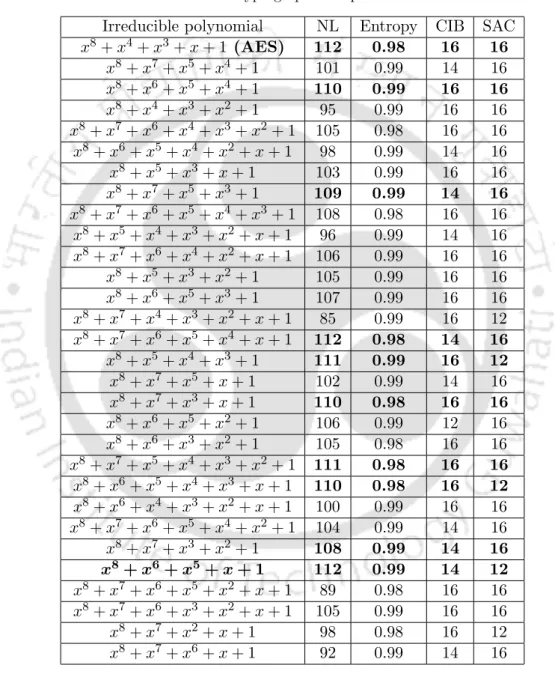Table 3.2: Values of Cryptographic Properties for AES S-Boxes Irreducible polynomial NL Entropy CIB SAC x 8 + x 4 + x 3 + x + 1 (AES) 112 0.98 16 16

## Construction of S-Box using Composite Field Arith- metic

First we need to map each element of GF(28) into its composite field with an isomorphic mapping function kef(q) = δ×q.

## Hardware Construction of CFA in Galois Field for S- Box

The GF((22)2) multiplier block is simplified by decomposing the field into GF((22)2) which implies two-bit instead of four-bit multiplications, as shown in Figure 3.4. Furthermore, it is simplified by decomposing the field into GF(22), where one-bit multiplication is performed.

## 2Xɸ

### Hardware Implementation of Composite Field Arith- metic

The theoretical CFA-based S-Box has been implemented and verified with a number of test vectors on FPGA (XC2VP30) Virtex-II pro board using Xilinx ISE tool, as shown in Table 3.5. The proposed architectural design of the CFA-based S-Box is implemented using Verilog for four optimal cases of {φ, λ} values, shown in Table 3.5 and Table 3.6.

Conclusion

### Box realization using Linear Cellular Automata and second

• Introduction
• Formulation of S-Box using Cellular Automata
• Proposed PCA based S-Box
• Performance comparison between conventional LUT S- Box and Dynamic PCA S-Box
• Formulation of S-Box using 2 nd order reversible one di- mensional cellular automata ( RCA 2 )
• Proposed RCA 2 based S-Box
• Security analysis of LUT and RCA 2 based S-Boxes
• Conclusion
• Introduction
• Proposed Hybrid Linear Cellular Automata (HLCA) based Encryption Algorithm architecture
• Comparison of hardware architecture and cryptographic properties
• Architecture of proposed HRCA 2 based encryption al- gorithm
• Comparison of Hardware Architecture and Security Anal- ysis
• Summary and conclusions
• Introduction
• Architecture of Camellia algorithm
• Proposed LPCA based F function
• Performance Comparison between LUT based S-Box of F function, LPCA based F function
• Proposed RCA 2 based F function
• Security analysis of LUT based S-Box of F function and RCA 2 based F function
• Summary
• Summary
• Contributions
• Directions for future work

The proposed architectural design of an S-Box based on an 8×8 PCA array implemented using logic gates, multiplexers and registers is shown in Figure 4.3. It is easily seen that the proposed PCA-based S-Box consumes 65% less energy than the existing work . The proposed RCA2-based S-Box architecture with AES algorithm has been implemented using Verilog and verified on an FPGA board.

From Table 4.6, it is clear that the proposed RCA2 based S-Box for AES algorithm exhibits a reduction in power dissipation and energy consumption compared to the existing works [88-93]. The security provided by the proposed HLCA algorithm has been validated using cryptographic properties as discussed in Section 3.4. The values ​​of SAC for the proposed HLCA-based encryption algorithm are shown in Figure 5.4.

The proposed HRCA2 encryption hardware requires some logic gates, registers and multiplexers, as shown in Figure 5.10. The level of security provided by the proposed HRCA2 algorithm against cryptanalysis was investigated using cryptographic properties as discussed in Section 3.4. The entropy values ​​for the proposed HRCA2-based encryption algorithm are plotted in Figure 5.12.

The security provided by the proposed HLCA and HRCA2 has been compared with the conventional AES algorithm. The security provided by the proposed RCA2-based F-function and LPCA-based F-function for the Camellia algorithm has been investigated using cryptographic properties. It has been observed that the proposed RCA2 and LPCA F-functions provide similar performance in terms of security compared to LUT-based S-Box ofF function for the Camellia algorithm.

The observed values ​​of entropy for the proposed F-function realization are plotted in Figure 6.11. The main contributions of the research work reported in this thesis include:. i) The construction of S-Box is achieved using various polynomial equations and the S-Box has been implemented using CFA technique. ii) The architectures of S-Box have been implemented on hardware using PCA and RCA2. iii). Based on the result of this thesis, this section provides the possible future directions for research. i) The CFA-based S-Box has been implemented on the FPGA.

Zhou, "The Automated Model for Cloud Storage," in 2012 International Conference on Information Science and Technology (ICIST), March 2012, pp.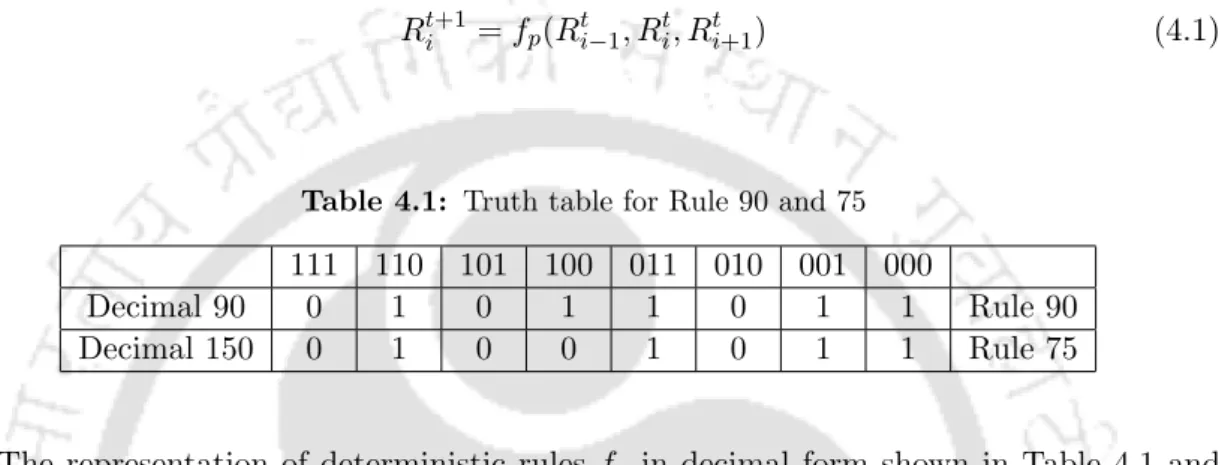Table 4.1: Truth table for Rule 90 and 75 111 110 101 100 011 010 001 000

Figure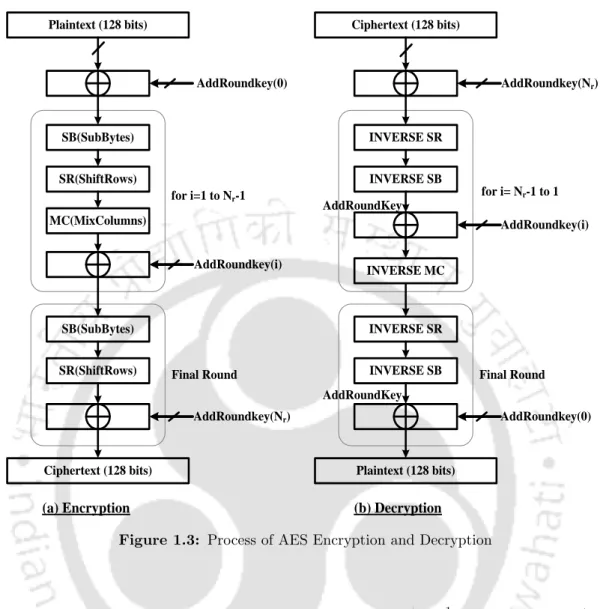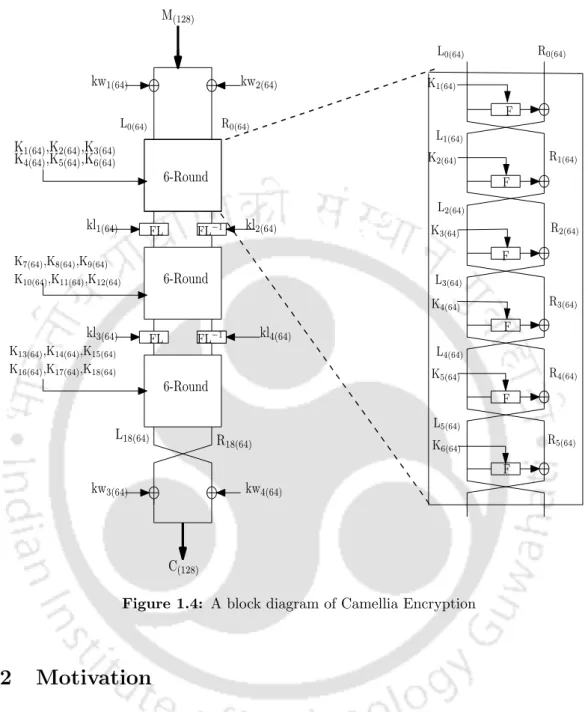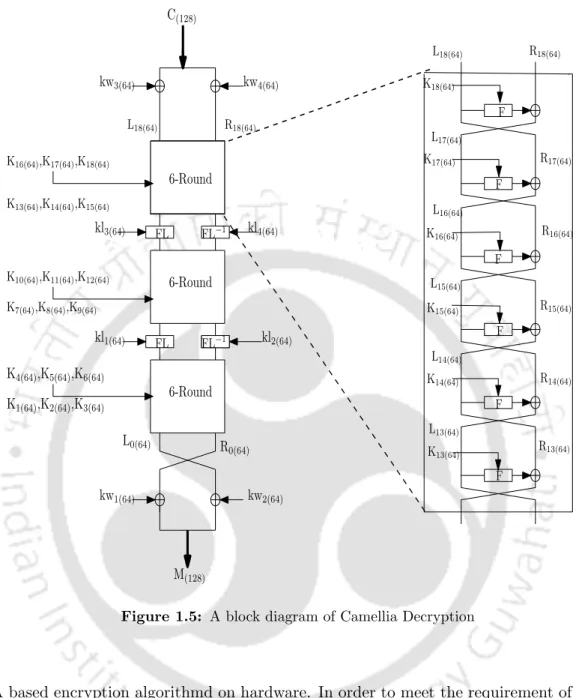+7

References

Related documents

2 hrs 2.5 Theory of organizational structures - nature and consequence of structure 2 hrs 3 Module B: Impact of structure, organization change and intervention strategy 3.1

4 Analysis of gaussian function An important property of gaussian function is that fourier transform of gaussian function is gaussian function in frequency domain.. Lets us consider

Invasive Fungal infection in Acute Myeloid Leukemia Associated with Myeloid sarcoma of Sinonasal Cavity a rare case – Indian Journal of Otolaryngology & Head & Neck Surgery National

Here a novel method is proposed that can be used during logic design to reduce the leakage power of CMOS circuits that use clock gating to reduce the dynamic power dissipation...

Given the alarming increase in the number of people with diabetes and shortage of trained retinal specialists and graders of retinal photographs, an automated approach involving a

EEE II-Sem L T P C 0 0 2 1 Prerequisite: Control Systems Course Objectives:  To understand the different ways of system representations such as Transfer function representation

ECE - I Semester T P C4+1 0 4EC 05399 MICROCONTROLLERS AND APPLICATIONS ELECTIVE – IUNIT IOVERVIEW OF ARCHITECTURE AND MICROCONTROLLER RESOURCES : Architecture of amicrocontroller –

Figure 5.6 Search domain: 0≤ ≤x 1 and 0≤ ≤y 1 a division of domain into 4 cells b fuzzification of function values 120 Figure 5.7 Reducing sizes of search domain and the optimum zone

L T P C 3 0 0 3 Prerequisite: Power System-I, Power System-II, Power System Protection, Power System Operation and Control, Power Electronics Course Objectives:  To compare EHV